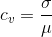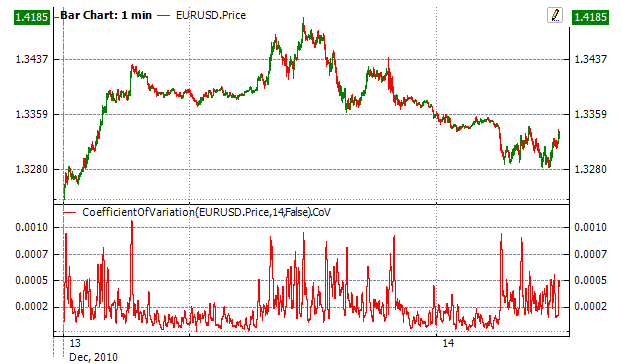﻿Coefficient Of Variation

# Coefficient Of Variation

The coefficient of variation (CoefficientOfVariation) (CoV), also known as relative standard deviation (RSD), is a standardized measure of dispersion of a probability distribution or frequency distribution. It is defined as the ratio of the standard deviation to the mean. When used in the stock market, it helps to determine the amount of volatility in comparison to the expected return rate of investment.CalculationChart ExampleImplementation and Usage

To initialize Coefficient Of Variation indicator, use one of the following constructors:

CoefficientOfVariation – set default values: period = 14

CoefficientOfVariation(Int32) – set value for period

CoefficientOfVariation(TimeSpan) – sets time period

Use

CoV - property to get current valueExample
C#
``` 1// Create new instance
2var indicator = new CoefficientOfVariation(28);
3
4// Number of stored values
5indicator.HistoryCapacity = 2;
6